ESTIMATE Statement

ESTIMATE ’label’ contrast-specification <(divisor=n)>
<, ’label’ contrast-specification <(divisor=n)>> <, …> </ options> ;

The ESTIMATE statement provides a mechanism for obtaining custom hypothesis tests. As in the CONTRAST statement, the basic element of the ESTIMATE statement is the contrast-specification, which consists of MODEL and RANDOM effects and their coefficients. Specifically, a contrast-specification takes the form

< fixed-effect values …> < | random-effect values …>

Based on the contrast-specifications in your ESTIMATE statement, PROC HPMIXED constructs the matrix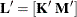, as in the CONTRAST statement, where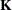is associated with the fixed effects and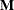is associated with the G-side random effects.

PROC HPMIXED then produces for each rowof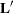an approximate t test of the hypothesis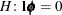, where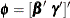. Results from all ESTIMATE statement are combined in the Estimates ODS table.

Note that multi-row estimates are permitted. Unlike the CONTRAST statement, you need to specify a ’label’ for every row of the multi-row estimate, since PROC HPMIXED produces one test per row.

PROC HPMIXED selects the degrees of freedom to match those displayed in the Type III Tests of Fixed Effects table for the final effect you list in the ESTIMATE statement. You can modify the degrees of freedom by using the DF= option. If you select DDFM=NONE and do not modify the degrees of freedom by using the DF= option, PROC HPMIXED uses infinite degrees of freedom, essentially computing approximate z tests.

If PROC HPMIXED finds the fixed-effects portion of the specified estimate to be nonestimable, then it displays Non-est for the estimate entry.

The construction of thematrix for an ESTIMATE statement follows the same rules as listed under the CONTRAST statement.

Table 46.5 summarizes the options available in the ESTIMATE statement.

Table 46.5: ESTIMATE Statement Options

Option

Description

Specifies the confidence level

Constructs t-type confidence limits

Specifies the degrees of freedom

Specifies values to divide the coefficients

Displays the matrix coefficients

Sets up random-effect contrasts between groups

Tunes the estimability checking

Sets up random-effect estimates between subjects

You can specify the following options in the ESTIMATE statement after a slash (/).

ALPHA=number

requests that a t-type confidence interval be constructed with confidence level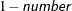. The value of number must be between 0 and 1 exclusively; the default is 0.05. If DDFM=NONE and you do not specify degrees of freedom with the DF= option, PROC HPMIXED uses infinite degrees of freedom, essentially computing a z interval.

CL

requests that t-type confidence limits be constructed. If DDFM=NONE and you do not specify degrees of freedom with the DF= option, PROC HPMIXED uses infinite degrees of freedom, essentially computing a z interval. The confidence level is 0.95 by default.

DF=number

specifies the degrees of freedom for the t-test. The default is the denominator degrees of freedom taken from the Type III Tests of Fixed Effects table and corresponds to the final effect you list in the ESTIMATE statement.

DIVISOR=value-list

specifies a list of values by which to divide the coefficients so that fractional coefficients can be entered as integer numerators. If you do not specify value-list, a default value of 1.0 is assumed. Missing values in the value-list are converted to 1.0.

If the number of elements in value-list exceeds the number of rows of the estimate, the extra values are ignored. If the number of elements in value-list is less than the number of rows of the estimate, the last value in value-list is copied forward.

If you specify a row-specific divisor as part of the specification of the estimate row, this value multiplies the corresponding divisor implied by the value-list. For example, the following statement divides the coefficients in the first row by 8, and the coefficients in the third and fourth row by 3:

estimate 'One vs. two'   A 2 -2  (divisor=2),
'One vs. three' A 1  0 -1         ,
'One vs. four'  A 3  0  0 -3      ,
'One vs. five'  A 1  0  0  0  -1  / divisor=4,.,3;
E

requests that the matrix coefficients be displayed. For ODS purposes, the name of this L Matrix Coefficients table is Coef.

GROUP coeffs

sets up random-effect contrasts between different groups when a GROUP= variable appears in the RANDOM statement. By default, ESTIMATE statement coefficients about random effects are distributed equally across groups. If you enter a multi-row estimate, you can also enter multiple rows for the GROUP coefficients. If the number of GROUP coefficients is less than the number of contrasts in the ESTIMATE statement, the HPMIXED procedure cycles through the GROUP coefficients. For example, the following two statements are equivalent:

estimate 'Trt 1 vs 2 @ x=0.4' trt 1 -1  0 | x 0.4,
'Trt 1 vs 3 @ x=0.4' trt 1  0 -1 | x 0.4,
'Trt 1 vs 2 @ x=0.5' trt 1 -1  0 | x 0.5,
'Trt 1 vs 3 @ x=0.5' trt 1  0 -1 | x 0.5 /
group 1 -1, 1 0 -1, 1 -1, 1 0 -1;

estimate 'Trt 1 vs 2 @ x=0.4' trt 1 -1  0 | x 0.4,
'Trt 1 vs 3 @ x=0.4' trt 1  0 -1 | x 0.4,
'Trt 1 vs 2 @ x=0.5' trt 1 -1  0 | x 0.5,
'Trt 1 vs 3 @ x=0.5' trt 1  0 -1 | x 0.5 /
group 1 -1, 1 0 -1;
SINGULAR=number

tunes the estimability checking as documented for the SINGULAR= in the CONTRAST statement.

SUBJECT coeffs

sets up random-effect estimates between different subjects when a SUBJECT= variable appears in the RANDOM statement. By default, ESTIMATE statement coefficients about random effects are distributed equally across subjects. Listing subject coefficients for an ESTIMATE statement with multiple rows follows the same rules as for GROUP coefficients.